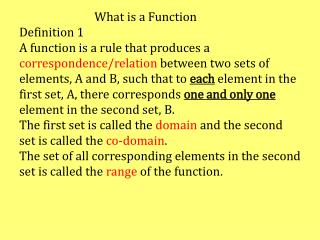DownloadDownload PresentationFunctions as Mapping Diagrams

# Functions as Mapping Diagrams

Télécharger la présentation## Functions as Mapping Diagrams

- - - - - - - - - - - - - - - - - - - - - - - - - - - E N D - - - - - - - - - - - - - - - - - - - - - - - - - - -
##### Presentation Transcript

1. What is a FunctionDefinition 1A function is a rule that produces a correspondence/relation between two sets of elements, A and B, such that to each element in the first set, A, there corresponds one and only one element in the second set, B.The first set is called the domain and the second set is called the co-domain. The set of all corresponding elements in the second set is called the range of the function.

2. Functions as Mapping Diagrams A B f a 2 b 4 c 6 d 8 Diagram 1 Does Diagram 1 represent a function? Yes, it does. Each element in the domain A is mapped to one and only one element in the co-domain B. NB: Co-domain ={2, 4, 6, 8} Range = {2,4, 6, 8}

3. Functions as Mapping Diagrams A B f a 2 b 4 c 6 d 8 Diagram 2 Does Diagram 2 represent a function? Yes, it does. Each element in the domain A is mapped to one and only one element in the co-domain B. NB: Co-domain ={2, 4, 6, 8} Range = {4, 6, 8} Since the element 2 in B is not mapped onto

4. Functions as Mapping Diagrams A B f a 2 b 4 c 6 d 8 Diagram 3 Does Diagram 3 represent a function? No, it does not. a 𝛜 A is not mapped to any element in B

5. Functions as Mapping Diagrams A B f 2 b 4 c 6 d 8 Diagram 4 Does Diagram 4 represent a function? No, it does not. b 𝛜 A is mapped to more than one element in B

6. Functions as Mapping Diagrams A B Function Notation Under the function, f, 6 is the image of c i.e. f(c)=6 Read as ‘f of c is 6’ 8 is the image of d i.e. f(d)=8 4 is the image of a and b i.e. f(a)=f(b)=4 Conversely, we can say that c is the pre-image of 6 f a 2 b 4 c 6 d 8 Diagram 1

7. State which of the following mappings represent functions. If the mapping represents a function state the domain, the co-domain and the range of the function. E C X X X D Y Y Y F g f f f f p s h n 1 1 1 1 b 2 i e u 4 2 2 a a 4 c a k 3 3 d d 9 t 4 4 16 e

8. X Y Can a function from X to Y be created if each element in Y is to be mapped onto? f 1 1 2 2 3 3 4

9. E F h Is the mapping h: E →F a function? If yes state the domain and the range of the function 2 2 3 4 4 6 No. 2 𝛜 E is not mapped to any element in F 5 8 Diagram 1 Show Answer

10. Is the mapping g: C →D a function? If yes state the domain and the range of the function C D g 2 2 3 4 4 Yes. Each element in C is mapped to an element in D Domain = {2, 3, 4, 5} Range = {2, 4, 6, 8} 6 5 8 Diagram 1 Show Answer

11. Is the mapping g: X →Y a function? If yes state the domain and the range of the function X Y g a 2 b 4 c 6 No. b 𝛜 X is mapped to more than one element in Y d 8 Diagram 1 Show Answer

12. Ordered Pairs A B f f can also be written as a set of ordered pairs where f={( , ), ( , ), ( , ), ( , ) 1 1 2 2 2 4 4 4 3 3 6 6 4 4 8 8 Diagram 1 Definition 2 A function is a set of ordered pairs with the property that no two ordered pairs have the same first component and different second components. The set of all first components in a function is called the domain of the function, and the set of all second components is called the range.

13. A B f Write the function f:A→B as a set of ordered pairs. a 1 b c 3 f={(a, 1), (b, 1), (c, 3), (d, 3)} d Diagram 2 Show Answer

14. State which of the following sets of ordered pairs represent a function. If it represents a function state the domain and the range of the function. f={(2,,4), (3, 6), (4, 8), (5, 10)} g={(1, 1), (1, 2), (2,, 2), (3, 3)} Show Answer Yes. No 2 ordered pairs have the same 1st component and different 2nd components Domain = {2, 3, 4, 5} Range = {4, 6, 8, 10} Show Answer No. The element 1 is mapped to more than 1 element.